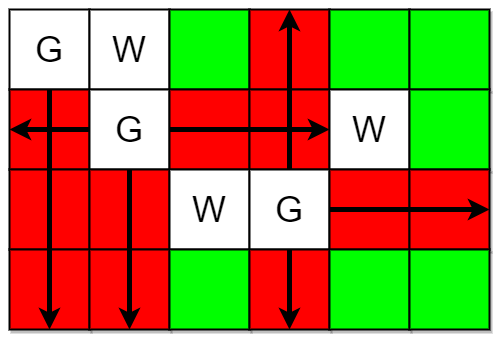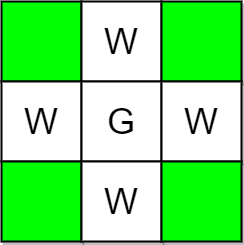2257. Count Unguarded Cells in the Grid
Medium
356
33

You are given two integers `m` and `n` representing a 0-indexed `m x n` grid. You are also given two 2D integer arrays `guards` and `walls` where `guards[i] = [rowi, coli]` and `walls[j] = [rowj, colj]` represent the positions of the `ith` guard and `jth` wall respectively.

A guard can see every cell in the four cardinal directions (north, east, south, or west) starting from their position unless obstructed by a wall or another guard. A cell is guarded if there is at least one guard that can see it.

Return the number of unoccupied cells that are not guarded.

Example 1:```Input: m = 4, n = 6, guards = [[0,0],[1,1],[2,3]], walls = [[0,1],[2,2],[1,4]]
Output: 7
Explanation: The guarded and unguarded cells are shown in red and green respectively in the above diagram.
There are a total of 7 unguarded cells, so we return 7.
```

Example 2:```Input: m = 3, n = 3, guards = [[1,1]], walls = [[0,1],[1,0],[2,1],[1,2]]
Output: 4
Explanation: The unguarded cells are shown in green in the above diagram.
There are a total of 4 unguarded cells, so we return 4.
```

Constraints:

• `1 <= m, n <= 105`
• `2 <= m * n <= 105`
• `1 <= guards.length, walls.length <= 5 * 104`
• `2 <= guards.length + walls.length <= m * n`
• `guards[i].length == walls[j].length == 2`
• `0 <= rowi, rowj < m`
• `0 <= coli, colj < n`
• All the positions in `guards` and `walls` are unique.
Accepted
13.7K
Submissions
26.3K
Acceptance Rate
52.0%

Seen this question in a real interview before?
1/4
Yes
No

Discussion (0)

Related Topics